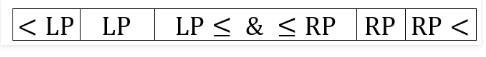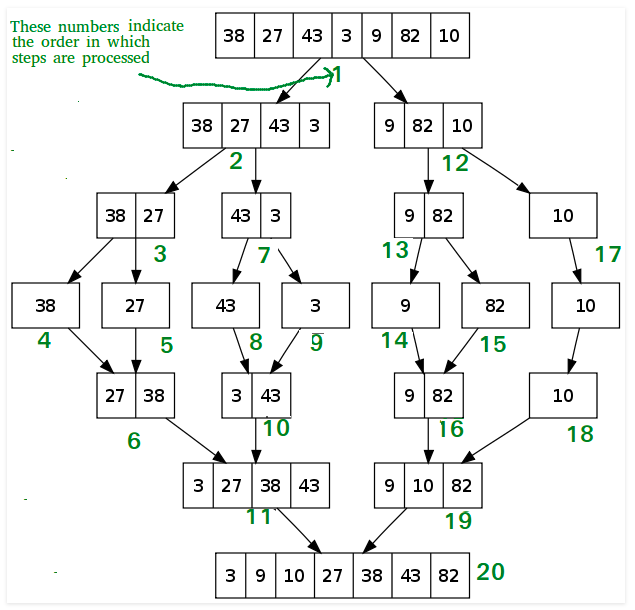# Quick Sort

In Arrays.sort(int[] a) of Java, it use the Dual-pivot Quick Sort algorithm:

## One-pivot Quick Sort

Quick Sort is a Divide and Conquer algorithm. It picks an element as pivot and partitions the given array around the picked pivot. There are many different versions of quick sort that pick pivot in different ways:

1. Always pick first element as pivot.
2. Always pick last element as pivot (implemented below)
3. Pick a random element as pivot.
4. Pick median as pivot.
 1234567891011121314151617181920212223242526272829303132333435363738394041424344454647 class QuickSort { /* This function takes last element as pivot, places the pivot element at its correct position in sorted array, and places all smaller (smaller than pivot) to left of pivot and all greater elements to right of pivot */ int partition(int arr[], int low, int high) { int pivot = arr[high]; int i = (low-1); // index of smaller element for (int j=low; j Array to be sorted, low --> Starting index, high --> Ending index */ void sort(int arr[], int low, int high) { if (low < high) { /* pi is partitioning index, arr[pi] is now at right place */ int pi = partition(arr, low, high); // Recursively sort elements before // partition and after partition sort(arr, low, pi-1); sort(arr, pi+1, high); } } } 

It’s an unstable and in-place sort algorithm, the time complexity can be expressed as the recurrence relation: $T(n) = T(k) + T(n-k-1) + n$

• In worst case, we get extremely unbalanced array after each partition: $T(n) = T(0) + T(n-1) + n = T(n-1) + n = n * (n + 1) /2 \approx O(n^2)$
• In best case, we get equally partitioned array each time: $$T(n) = 2*T(n/2)+n = 2*(T(n/4)+n/2)+n = 2*(2*(T(n/8)+n/4)+n/2)+n = 2^k*T(n/2^k)+k*n$$ When $k = \log_2 n, T(n/2^k) = T(1), T(n) = C*n + n * \log_2 n \approx O(n \log n)$
• In general case, we can suppose the partition puts O(n/9) elements in one set and O(9n/10) elements in other set: $T(n) = T(n/9) + T(n/10) + n \approx O(n \log n)$

quick-sort

## Dual-pivot Quick Sort

The idea of dual pivot quick sort is to take two pivots, one in the left end of the array and the second, in the right end of the array. The left pivot must be less than or equal to the right pivot, so we swap them if necessary. Then, we begin partitioning the array into three parts: in the first part, all elements will be less than the left pivot, in the second part all elements will be greater or equal to the left pivot and also will be less than or equal to the right pivot, and in the third part all elements will be greater than the right pivot. Then, we shift the two pivots to their appropriate positions as we see in the below bar, and after that we begin quick sorting these three parts recursively, using this method.Dual pivot quick sort is a little bit faster than the original single pivot quicksort. But still, the worst case will remain $O(n^2)$ when the array is already sorted in an increasing or decreasing order.

dual-pivot-quicksort

# Merge Sort

In Arrays.sort(Object[] a) of Java, it use the Merge Sort algorithm. Like QuickSort, Merge Sort is a Divide and Conquer algorithm. It divides input array in two halves, calls itself for the two halves and then merges the two sorted halves. The merge(arr, l, m, r) is key process that assumes that arr[l..m] and arr[m+1..r] are sorted and merges the two sorted sub-arrays into one.123456789101112131415161718192021222324252627282930313233343536373839404142434445464748495051525354555657585960616263646566 class MergeSort { // Merges two subarrays of arr[]. // First subarray is arr[l..m] // Second subarray is arr[m+1..r] void merge(int arr[], int l, int m, int r) { // Find sizes of two subarrays to be merged int n1 = m - l + 1; int n2 = r - m; /* Create temp arrays */ int L[] = new int [n1]; int R[] = new int [n2]; /*Copy data to temp arrays*/ for (int i=0; i

It’s a stable but not in-place sort algorithm, the time complexity can be expressed as following recurrence relation in best, worst, average cases:

$$T(n) = 2*T(n/2)+n = 2*(T(n/4)+n/2)+n = 2*(2*(T(n/8)+n/4)+n/2)+n = 2^k*T(n/2^k)+k*n$$ The space complexity is O(n), but in case of sorting linked list, the space complexity is O(1):

 1234567891011121314151617181920212223242526272829303132333435363738394041424344454647484950515253545556575859606162636465666768 public class linkedList { node head = null; // node a, b; static class node { int val; node next; public node(int val) { this.val = val; } } node sortedMerge(node a, node b) { node result = null; /* Base cases */ if (a == null) return b; if (b == null) return a; /* Pick either a or b, and recur */ if (a.val <= b.val) { result = a; result.next = sortedMerge(a.next, b); } else { result = b; result.next = sortedMerge(a, b.next); } return result; } node mergeSort(node h) { // Base case : if head is null if (h == null || h.next == null) { return h; } // get the middle of the list node middle = getMiddle(h); node nextofmiddle = middle.next; // set the next of middle node to null middle.next = null; // Apply mergeSort on left list node left = mergeSort(h); // Apply mergeSort on right list node right = mergeSort(nextofmiddle); // Merge the left and right lists node sortedlist = sortedMerge(left, right); return sortedlist; } // Utility function to get the middle of the linked list node getMiddle(node h) { // Base case if (h == null) return h; node fastptr = h.next; node slowptr = h; // Move fastptr by two and slow ptr by one // Finally slowptr will point to middle node while (fastptr != null) { fastptr = fastptr.next; if (fastptr != null) { slowptr = slowptr.next; fastptr = fastptr.next; } } return slowptr; } } 

merge-sort